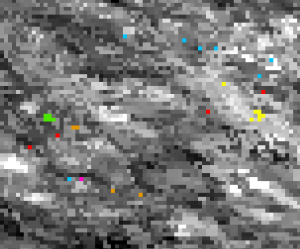# Image classification with Pythonm

#### March 3, 2016

We are going to classify a multitemporal image stack of MODIS NDVI time series (MOD13Q1). The stack consists of 23 bands (16-day composites) with a spatial resolution of 231m in sinusoidal projection. We want to classify the different land use types, especially to discriminate different crop types.

Install Python and required image processing and scientific programming packages:

`sudo apt-get install python-numpy scikit-learn scikit-image`

For beginners, the basic Python IDLE is sufficient for scripting. However, another IDE, LiClipse is highly recommended: http://www.liclipse.com/

We assume that you already have created a bunch of training samples in 8bit TIFF format with distinct class labeling (1,2,3,4, etc). It is necessary that these single pixels are snapped to the pixel size of the original dataset and have the same dimensions and extent.

Now write your Training script:

```# import all required Python packages:
import skimage.io as io
import numpy as np
import os, shutil

from sklearn.ensemble import AdaBoostClassifier, RandomForestClassifier, GradientBoostingClassifier, ExtraTreesClassifier
from sklearn.externals import joblib

# set up your directories with the MODIS data
rootdir = "C:\\Data\\Raster\\MODIS\\"
# path to your training data
path_pix = "C:\\Data\\Samples\\"
# path to your model
path_model = "C:\\Data\\Models\\"
# path to your classification results
path_class = "C:\\Data\\Class\\"

# declare a new function
def training():
# path to your MODIS TIFF
raster = rootdir + "modis_stack_ndvi.tif"
# path to your corresponding pixel samples (training data)
samples = path_pix + "samples_modis.tif"

# read in MODIS raster
# convert to 16bit numpy array
img = np.array(img_ds, dtype='int16')

# do the same with your sample pixels
roi = np.array(roi_ds, dtype='int8')

labels = np.unique(roi[roi > 0])
print('The training data include {n} classes: {classes}'.format(n=labels.size, classes=labels))

# compose your X,Y data (dataset - training data)
X = img[roi > 0, :]
Y = roi[roi > 0]

# assign class weights (class 1 has the weight 3, etc.)
weights = {1:3, 2:2, 3:2, 4:2}

# build your Random Forest Classifier

rf = RandomForestClassifier(class_weight = weights, n_estimators = 100, criterion = 'gini', max_depth = 4,
min_samples_split = 2, min_samples_leaf = 1, max_features = 'auto',
bootstrap = True, oob_score = True, n_jobs = 1, random_state = None, verbose = True)

# alternatively you may try out a Gradient Boosting Classifier
# It is much less RAM consuming and considers weak training data
"""
rf = GradientBoostingClassifier(n_estimators = 300, min_samples_leaf = 1, min_samples_split = 4, max_depth = 4,
max_features = 'auto', learning_rate = 0.8, subsample = 1, random_state = None,
warm_start = True)
"""

# now fit your training data with the original dataset
rf = rf.fit(X,Y)

# export your Random Forest / Gradient Boosting Model
model = path_model + "model.pkl"
joblib.dump(rf, model)

training()```

And now write your classification script:

```def classification():
# Read worldfile of original dataset
tfw_old = str(raster.split(".tif")) + ".tfw"

img = np.array(img_ds, dtype='int16')

# call your random forest model
rf = path_model + "model.pkl"

# Classification of array and save as image (23 refers to the number of multitemporal NDVI bands in the stack)
new_shape = (img.shape * img.shape, img.shape)
img_as_array = img[:, :, :23].reshape(new_shape)

class_prediction = clf.predict(img_as_array)
class_prediction = class_prediction.reshape(img[:, :, 0].shape)

# now export your classificaiton
classification = path_class  + "classification.tif"
io.imsave(classification, class_prediction)

# Assign Worldfile to classified image
tfw_new = classification.split(".tif") + ".tfw"
shutil.copy(tfw_old, tfw_new)

classification()```

Multitemporal NDVI MODIS Stack with clipped AOI:Training pixels with distinct classes are created from field sampling points and snapped to the original raster extent:Classification results (left image: same extent as above):

you may also like:

most recent news: